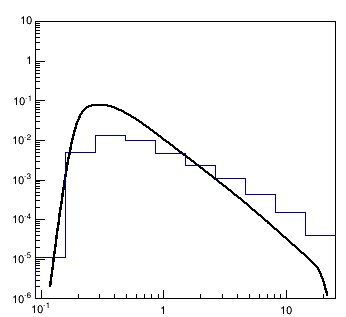# TF1 random number generator

Hi everyone,

Look at the attached plot. I am given a TF1 function which I use to generate random number and fill them into histogram. But they don’t quite match.

Regards

``````  int nb = 10;  // number of bins in hist in log scale
double factor = pow(10,log10(25/0.09)/10);
double bins[ nb+1 ]; // making equal bin width in log scale
int i;  double myX;
int off_events = 10000;
bins[ 0 ] = 0.09;
for( i = 1; i <= nb; i++ )
{
bins[ i ] = bins[ i-1 ] * factor;
}
TH1F *off_data = new TH1F( "off","Off data" ,nb, bins );
for( i = 0; i < off_events; i++ )
{
myX = f_Conv->GetRandom();  // this is a tf1
off_data->Fill( myX );
}
for( i = 0; i <= nb; i++ )
{
myX = off_data->GetBinContent(i)*tflux/off_events;
// tflux is integral of tf1
off_data->SetBinContent( i, myX );  // renormalizing
}
f_Conv->Draw();
off_data->Draw("same");